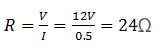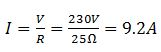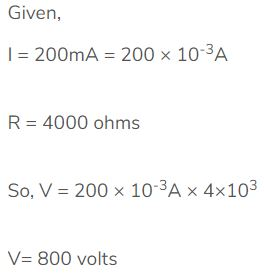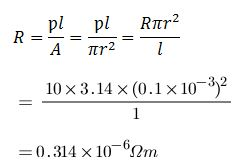## Solutions of Electricity Lakhmir Singh Manjit Kaur VSAQ, MCQ and HOTS Pg No. 19 Class 10 Physics20A. What is the ratio of potential difference and current known as?

→ Resistance is the ratio of potential difference across a component to the current flowing through it, it is measure in ohms.

20B. The values of potential difference V applied across a resistor and the corresponding values of current I flowing in the resistor are given below :
Potential difference, V (in volts):2.55.010.015.020.025.0
Current, I (in amperes)0.10.20.40.60.81.0

Plot a graph between V and I, and calculate the resistance of the resistor.

→ The plot between voltage and current is called IV characteristics. The voltage is plotted on y- axis. The values of the current for different values of the voltage are shown in the given table
The plot between voltage and current is called IV characteristic. The voltage is plotted on x-axis and current is plotted on y-axis. The values of the current for different values of the voltage are shown in the given table.20C. Name the law which illustrated by the above V-I graph.

→ From these values we conclude that ration V/I is a constant i.e., V α I. It means that Ohm’s law is being followed.

20D. Write down the formula which states the relation between potential difference, current and resistance.

→ The formula is given by Ohm which states the relation between potential difference, current and resistance.
Potential difference = Current × Resistance

20E. The potential difference between the terminals of an electric iron  is 240 V and the current is 5.0 A. What is the resistance of the electric iron?

→ Here, V=240 volt, I= 5 A, R=?
According to Ohms Law:-V= IRMultiple Choice Questions (MCQs)-Pg-19

21. The p.d. across a 3Ω resistor is 6 V. The current flowing in the resistor will be:
A. 5A
B. 1A
C. 2A
D. 6A

→ As we know that22. A car headlight bulb working on a 12 V car battery draws a current of 0.5 A. The resistance of the light bulb is:
A. 0.5 Ω
B. 6 Ω
C. 12 Ω
D. 24 Ω

→ As we know that23. An electrical appliance has a resistance of 25 Ω. When this electrical appliance is connected to a 230 V supply line, the current passing through it will be :
A. 0.92 A
B. 2.9 A
C. 9.2 A
D. 92 A24. When a 4Ω resistor is connected across the terminals of a 12 V battery, the number of coulombs passing through the resistor per second is :
A. 0.3
B. 3
C. 4
D. 12

→ R= 4 ohms
V= 12 V
I= 12/4 =3A
I=Q/t
Q=3 C

25. Ohm’s law gives a relationship between:
A. current and resistance
B. resistance and potential difference
C. potential difference and electric charge
D. current and potential difference

→ Ohm’s law gives a relationship between current and potential difference.

26. The unit of electrical resistance is :
A. ampere
B. volt
C. coulomb
D. ohm

→ Ohm is the S.I unit of electrical resistance.

27. The substance having infinitely high electrical is called:
A. conductor
B. resistor
C. superconductor
D. insulator

→ The substance having infinitely high electrical is called conductor.

28. Keeping the potential difference constant, the resistance of a circuit is halved. The current will become:
A. one-fourth
B. four times
C. half
D. double

→ Current is inversely proportional to resistance.

29. Keeping the potential difference constant, the resistance of a circuit is halved. The current will become:
A. one-fourth
B. four times
C. half
D. double

→ Double

Questions Based on High Order Thinking Skills (HOTS)-Pg-20

30. An electric room heater draws a current of 2.4 A from the 120 V supply line. What current will this room heater draw when connected to 240 V supply line?

→ I=2.4A
potential difference = 120V:
R= V/I
R= 120/2.4 =50 ohms
Since resistance remains constant therefore if potential difference = 240 V

then, I= V/R = 240/50 = 4.8 A

31. Name the electrical property of a material whose symbol is “omega”.

→ The electrical property of a material whose symbol is “omega is resistance.

32. The graph between V and I for a conductor is a straight line passing through the origin.
(a) Which law is illustrated by such a graph?
(b) What should remain constant in a statement of this law?

→ (a) The I-V graph of the metallic conductor is a straight line pass through the origin. They obey Ohm’s Law, having a resistance that is independent of current. (Ohmic conductors). The variation of current vs voltage is shown below:(b) Temperature remains constant in ohms law.

33. A p.d. of 10 V is needed to make a current of 0.02 A flow through a wire. What p.d. is needed to make a current of 250 mA flow through the same wire?

→ Here I1 = 0.02 amp, V1 = 10 volt
V=IRAgain
Here I2 = 250mA=0.25A, R = 500
V2=I2R =0.25×500=125V

34. A current of 200 mA flows through a 4 KΩ resistor. What is the p.d. across the resistor?1. What happens to the resistance as the conductor is made thicker?

→ The resistance of the wire will decrease if we made the conductor thicker because R (resistance) = p (rho). l (length) / A (area of cross-section or thickness of wire). Area is directly proportional to the radius of the wire.

2. If the length of a wire is doubled by taking more of wire, what happens to its resistance?

→ As the resistance of a wire is directly proportional to length of the conducting wire. If its length is doubled resistance also doubled.

3. On what factors does the resistance of a conductor depend?

→ There are four factors that influence the resistance in a conductor. Thickness (cross sectional area of the wire), length, and temperature. The fourth factor is the conductivity of the material that is used.

4. Name the material which is the best conductor of electricity.

→ Silver is the best conductor of electricity.

5. Which among iron and mercury is a better conductor of electricity?

→ Mercury is a better conductor than iron as it has lower resistivity than iron.

6. Why are copper and aluminium wires usually used for electricity transmission?

→ Copper and aluminium wires usually used for electricity transmission because copper and aluminium are very good conductors of electricity having very low electric resistivity (or resistance).

7. Name the material which is used for making the heating element of an electric iron.

→ Nichrome wire is used as a heating element because it is very stable, even at high temperatures.

8. What is nichrome? State its one use.

→ Nichrome (NiCr, nickel-chrome, chrome-nickel, etc.) generally refers to any alloy of nickel, chromium, and often iron and/or other elements or substances. Nichrome alloys are typically used in resistance wire. They are also used in some dental restorations (fillings) and in other applications.

9. Give two reasons why nichrome alloy is used for making the heating elements of electrical appliances.

→ Firstly nicrome is an alloy, it has a higher resistivity and consequently a higher resistance.Therefore, it will resist the flow of charges more and lead to development of heat faster.
Secondly alloys like nichrome don't oxidise,i.e burn easily at high temperatures.

10. Why are the coils of electric irons and electric toasters made of an alloy rather than a pure metal?

→ The resistivity of an alloy is generally higher than that of its constituent metals. Alloys do not oxidise readily at high temperatures.

11. Which has more resistance:
(a) a long piece of nichrome wire or a short one?
(b) a thick piece of nichrome wire or a thin piece?

→ (a) The resistance of a wire depends on its dimensions as well as on the conducting ability of the material from which it is made. A long wire has more resistance than a short one.
(b) A thin wire has more resistance than a thick one.

12A. How does the resistance of a pure metal change if its temperature decreases?

→ If temperature of a metal decrease the resistance will also decrease.

12B. How does the presence of impurities in a metal affect its resistance?

→ The presence of impurities in a metal increase the resistance of the metal.

13. Fill in the following blanks with suitable words :
Resistance is measured in ............. The resistance of a wire increases as the length ...............; as the temperature ................; and as the cross-sectional area ............

→ Resistance is measured in Ohms. The resistance of a wire increases as the length increases, as the temperature increases, and as the cross-sectional area decreases.

14A. What do you understand by the "resistivity" of a substance?

→ The electrical resistivity of a material is also known as its specific electrical resistance. It is a measure of how strongly a material opposes the flow of electric current. A definition of resistivity is the electrical resistance per unit length and per unit of cross-sectional area.

14B. A wire is 1.0 m long, 0.2 mm in diameter and has a resistance of 10Ω. Calculate the resistivity of its material?

→ Given length of wire,l=1m.
Diameter of wire,D = 0.2mm∴ Radius of Wire,r=0.1mm= 0.1x10-3 m.15A. Write down an expression for the resistance of a metallic wire in terms of the resistivity.

→ Resistance of a metallic wire with resistivity  of length l and cross sectional area A is15B. What will be the resistance of a metal wire of length 2 metres and area of cross-section 1.55 x 10-6 m2, if the resistivity of the metal be 2.8 x 10-8 Ω m?16A. Give two examples of substances which are good conductors of electricity. Why do you think they are good conductors of electricity?

→ Metals are typically good conductors because of their ability to donate electrons. Most metal atoms have one or two valence electrons, which means that they want to give these away in order to become more stable. Silver and copper are good conductors of electricity because they have free electrons available for conduction.

16B. Calculate the resistance of a copper wire 1.0 km long and 0.50 mm diameter if the resistivity of copper is 1.7×10-8 Ω m.

→ According to Question,
Length of Wire= 1km=1000m
Diameter of Wire=0.50mm
Resistivity(ρ)=1.7x10-8Ωm17. Will current flow more easily through a thick wire or a thin wire of the same material when connected to the same source? Give reason for your answer.

→ The current will flow more easily through thick wire. It is because the resistance of a conductor is inversely proportional to its area of cross - section. If thicker the wire, less is resistance and hence more easily the current flows.

18. How does the resistance of a conductor depend on :
(a) length of the conductor?
(b) area of cross-section of the conductor?
(c) temperature of the conductor?

→ a) Resistance of a conductor is directly propotional to the length of conductor.
b) Resistance of a conductor is inversely proportional to the area of cross section of the conductor.
c) Resistance of a conductor depends upon the nature of the material of the conductor. Resistance of a conductor increases on raising the temperature and decreases on lowering the temperature.

19A. Give one example to show how the resistance depends on the nature of material of the conductor.

→ If we connect different type of wire (nichrome, copper etc) in ac ircuit made using a battery, ammeter. We will see that the ammeter shows different reading in each case i.e. resistance differes as nature of material changes.

19B. Calculate the resistance of an aluminium cable of length 10 km and diameter 2.0 mm if the resistivity of aluminium is 2.7 x 10-8Ωm.20. What would be the effect on the resistance of a metal wire of :
(a) increasing its length?
(b) increasing its diameter?
(c) increasing its temperature?

→ (a) The resistance of a metal wire will increase as it is directly proportional to length.
(b) The resistance of a metal wire decrease as it is inversely proportional to the area of the wire.
(c) Resistance of a conductor depends upon the nature of the material of the conductor. Resistance of a conductor increases on raising the temperature and decreases on lowering the temperature.

21. How does the resistance of a wire vary with its :
(a) area of cross-section?
(b) diameter?

→ (a) The resistance of a metal wire is inversely proportional to the area of the wire, so if the area increase resistance decrease.
(b) If diameter increase than area increase and the resistance decreases.

22. How does the resistance of a wire change when:
(i) its length is tripled?
(ii) its diameter is tripled?
(iii) its material is changed to one whose resistivity is three times?

→ (i) The resistance of a wire is directly proportional to its length and inversely proportional to its area (or square of inverse of diameter). When the length is tripled then its resistance will also becomes three times
(ii) If the diameter get tripled then its resistance becomes one ninth of the initial resistance.
(iii) If the material is changed to one whose resistivity is three times then again its resistance will be three times.

23. Calculate the area of cross section of a wire if its length is 1.0 m, its resistance is 23Ω and the resistivity of the material of the wire is 1.84 x 10-6 Ωm.